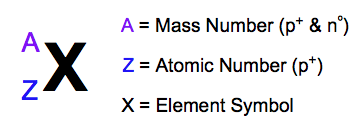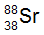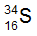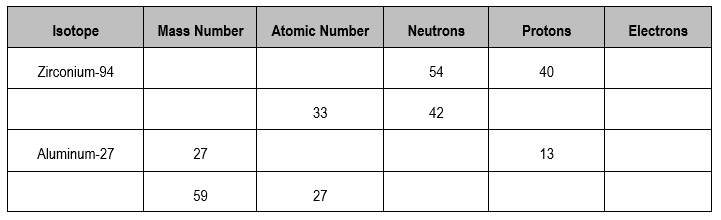Clutch Prep is now a part of Pearson
Ch.2 - Atoms & ElementsWorksheetSee all chapters

# Isotopes

See all sections
Sections
The Atom
Subatomic Particles
Isotopes
Ions
Atomic Mass
Periodic Table: Classifications
Periodic Table: Group Names
Periodic Table: Representative Elements & Transition Metals
Periodic Table: Element Symbols
Periodic Table: Elemental Forms
Periodic Table: Phases
Periodic Table: Charges
Calculating Molar Mass
Mole Concept
Law of Conservation of Mass
Law of Definite Proportions
Atomic Theory
Law of Multiple Proportions
Millikan Oil Drop Experiment
Rutherford Gold Foil Experiment

Isotopes are atoms of an element that have the same atomic number, but different mass number.

###### The Atomic View of Isotopes

Concept #1: Isotopes

Isotopes are elements with the same number of protons, but different number of neutrons.

Example #1: Calcium-43 represents one of the isotopes for the calcium atom. If the number 43 represents its mass number, determine the correct numbers for its subatomic particles.Practice: Which of the following answers give the correct number of subatomic particles for?

Practice: Which of the following answers give the correct number of subatomic particles for?

Practice: Fill in the gaps for the following table of atoms.